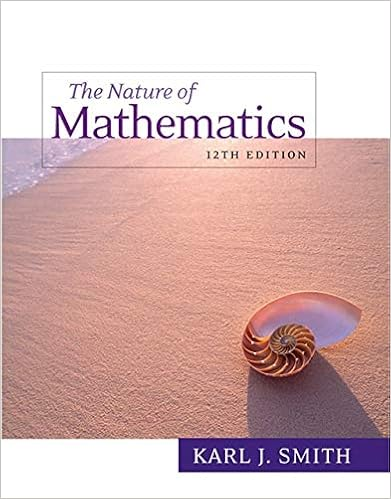# MA 114 FinalReview - Final Review MA 114-004 Fall 2015 The...

• Test Prep
• 9

This preview shows page 1 - 5 out of 9 pages.

##### We have textbook solutions for you!
The document you are viewing contains questions related to this textbook.The document you are viewing contains questions related to this textbook.
Chapter 1 / Exercise 9
Nature of Mathematics
SmithExpert Verified
Final Review MA 114-004 Fall 2015 The Final will cover will cover sections 1.1-1.4, 2.1-2.5, 3.1-3.2, 4.1-4.3, 4.5, 5.1- 5.7, 6.1-6.5 and 8.1-8.2 in Finite Mathematics and its Applications, Goldstein, Schneider, and Siegel, 11th Edition. Topics Equations of lines Graphing lines Graphing linear inequalities Finding feasible set of a system of linear inequalities Finding the intersection point of a pair of lines Addition Method Substitution Method Gaussian and Gauss-Jordan elimination Solving systems of at least 2 variables using the Gauss-Jordan method. Matrix inverse Finding inverse of 2 × 2 matrix Finding inverse of larger dimension using the Gauss-Jordan method Determining if an inverse exists Linear Programming Finding optimal solution using the steps outlined in class Definition of a simplex tableau Introduction of slack variables Solving optimization problems using the Simplex Method For problems in standard form For maximum problems 1
##### We have textbook solutions for you!
The document you are viewing contains questions related to this textbook.The document you are viewing contains questions related to this textbook.
Chapter 1 / Exercise 9
Nature of Mathematics
SmithExpert Verified
For minimum problems Duality Union and intersection of a sets Listing all subsets of a set Complement of a set The inclusion-exclusion principle DeMorgan’s Laws Venn diagram representation of sets The Multiplication Principle Permutations and combinations Formulas In applications Problems counting possible combinations with ‘or’ The Binomial Theorem Formula for a binomial coefficient Probability definitions experiment outcome sample space event mutually exclusive Determining a sample space Probability rules Computing probability when outcomes are equally likely Inclusion-Exclusion Rule Conditional probability Independence Tree Diagrams 2
Constructing Computing conditional probability and probability of an intersection Markov processes Transition stochastic matrices Properties of stochastic matrices Transition diagrams Computing probability distribution for all states Regular stochastic matrices Properties of the stable matrices Finding the stable matrix/distribution. 3
Some Practice Problems
•••